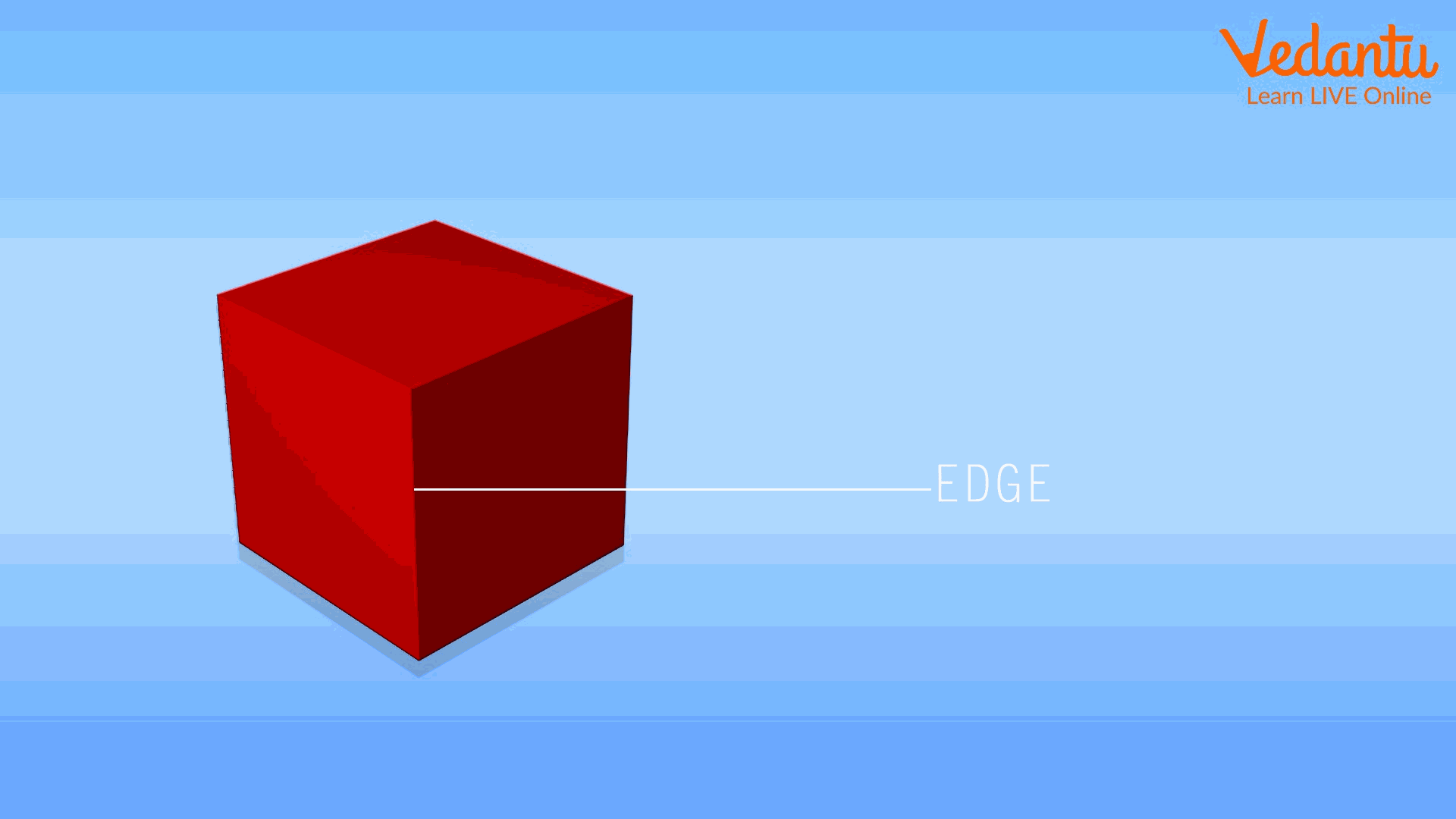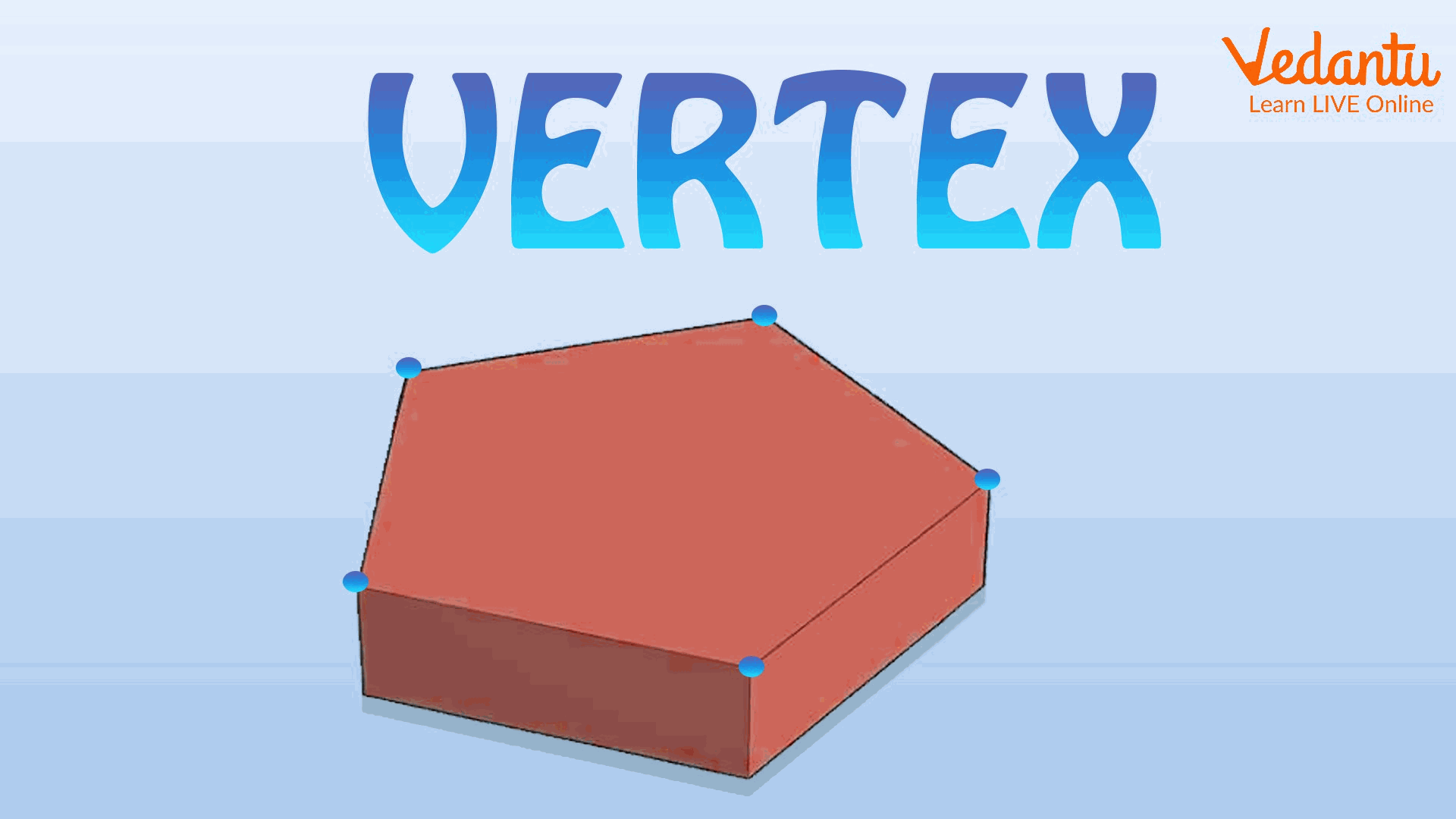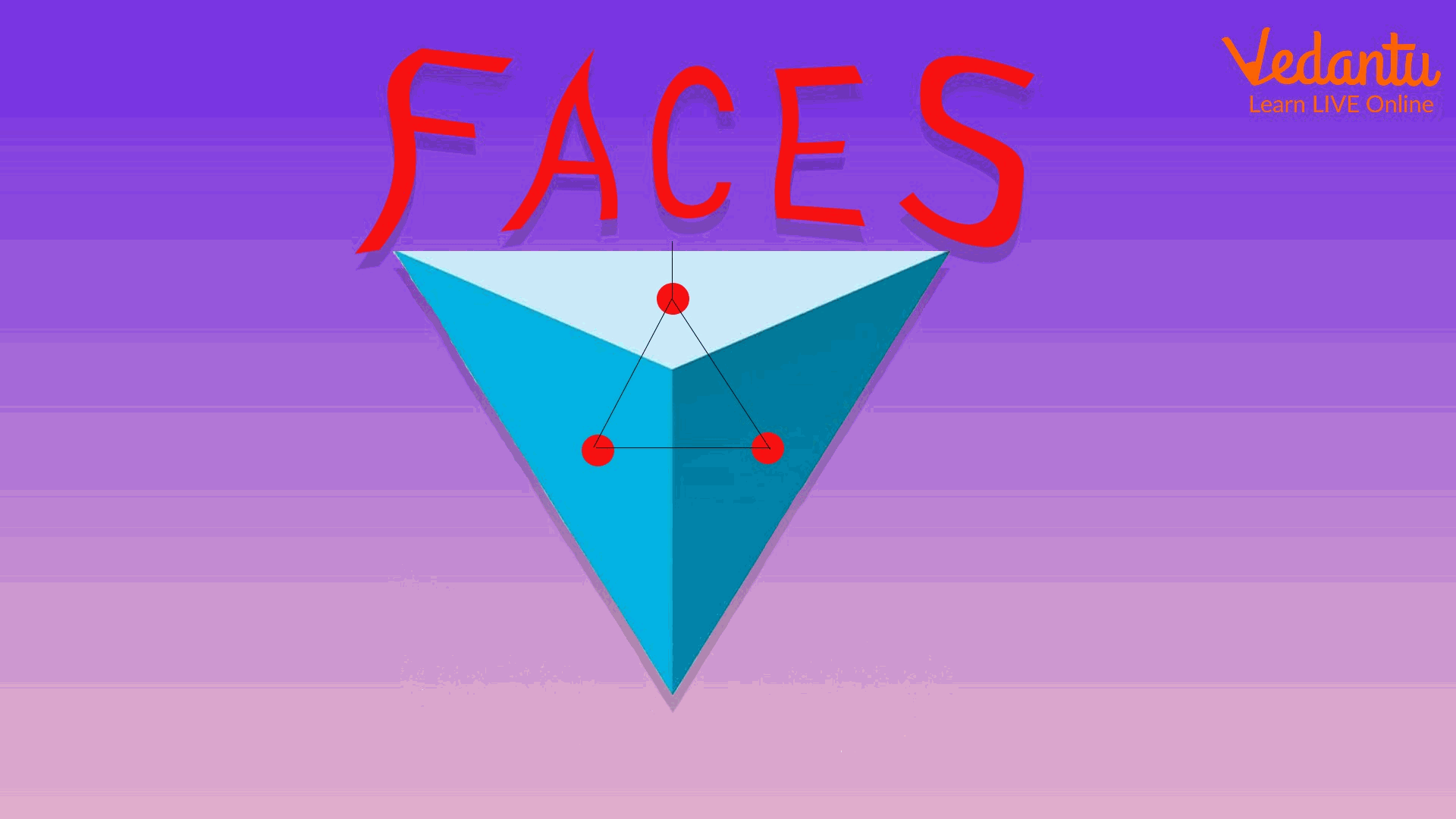Courses
Courses for Kids
Free study material
Offline Centres
More

# What are Edges, Vertices & Faces and Euler’s FormulaLast updated date: 03rd Dec 2023
Total views: 147.9k
Views today: 3.47k## What are Edges?

We see a number of things of different sizes and shapes in our day to day life such as ice-cream cones, balls, boxes and door mats etc. These objects are identified by different characteristic properties. These properties are length, breadth, diameter, perimeter, vertex, edges, faces etc. Edges of any shape are defined as “The line segment that joins two vertices and it acts as an interface between two faces.” In the following diagram, an edge of a square is shown.Edge of a Square

## What are Vertices and Faces?

A vertex of any shape is a point of contact or meeting point of two or more edges. Hence we can say that vertices are the corner points of a shape. The vertex of a pentagon is shown in the figure below.Vertices of a Pentagon

.

The face of any solid object is any of the individual flat surfaces. The tetrahedron has four faces which are shown below. One face you can not see in the 2-D figure.Faces of a Tetrahedron

## Euler's Formula

The relationship between vertices, edges and faces is given by Euler’s Formula. The Euler’s Formula for many solid shapes is given as : F + V − E = 2

Where F = Number of Faces

V= Number of Vertices

E= Number of Edges

Let’s apply this formula to the cube to verify it. We know that a cube has 6 faces, 8 vertices, and 12 edges

So F=6, V=8 and E=12 then putting these values in the equation we get,

6 + 8 − 12 = 2

2 = 2

Hence the formula is verified.

## Numbers of Edges, Vertices and Faces in Different Solids

The number of edges, vertices and faces in different three-dimensional solids are given below.

 Solid Name Vertices Faces Edges Cube 8 6 (faces are square) 12 Cuboid 8 6 (faces are rectangular) 12 Cone 1 2 1 Cylinder 0 3 2 Sphere 0 1 0 Tetrahedron 4 4 6

## Conclusion

After reading the above article students will learn basic about what are edges, vertices and faces of any solid. We also learned an interesting relationship between the three of them by learning Euler's formula. This formula holds good for closed solids which have flat faces and straight edges but cannot be used for cylinders as they have curved edges.

## FAQs on What are Edges, Vertices & Faces and Euler’s Formula

1. What are edges, vertices and faces?

Edges, vertices and faces are the three important properties found in any three-dimensional solids that are useful in characterizing and identifying a particular solid. An edge is a straight line between two faces, a vertex is the corner of the shape whereas a face is a flat surface. Different shapes of three-dimensional objects used to have different numbers of edges, vertices and faces.

2. How many edges, vertices and faces are found in a triangular prism?

A triangular prism in geometry is a solid prism containing three-sides. A triangular prism is a polyhedron that contains a triangular base and three faces joining corresponding sides. It has five faces, six vertices and nine edges. Hence the two faces of this polyhedron are parallel, while the surface normals of the other three are in the same plane. These three faces are parallelograms.